# 3D plotting#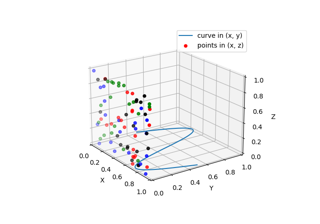Plot 2D data on 3D plot

Plot 2D data on 3D plot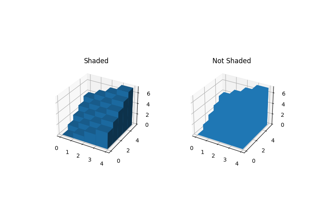Demo of 3D bar charts

Demo of 3D bar charts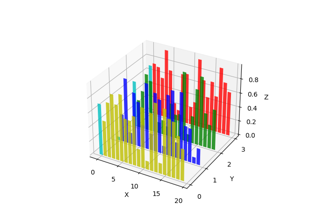Create 2D bar graphs in different planes

Create 2D bar graphs in different planes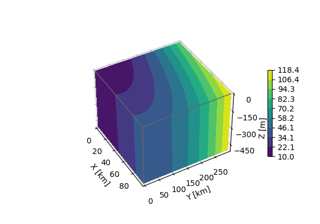3D box surface plot

3D box surface plot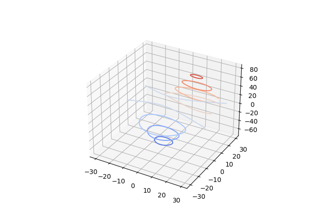Demonstrates plotting contour (level) curves in 3D

Demonstrates plotting contour (level) curves in 3D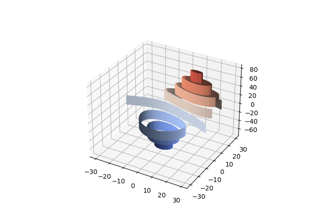Demonstrates plotting contour (level) curves in 3D using the extend3d option

Demonstrates plotting contour (level) curves in 3D using the extend3d option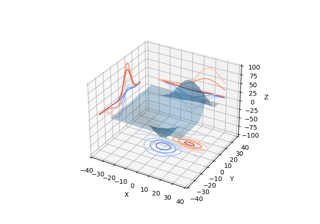Projecting contour profiles onto a graph

Projecting contour profiles onto a graph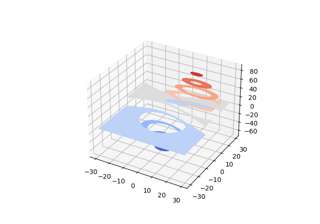Filled contours

Filled contours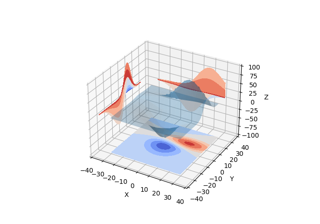Projecting filled contour onto a graph

Projecting filled contour onto a graph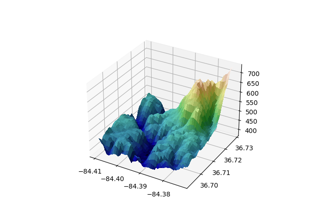Custom hillshading in a 3D surface plot

Custom hillshading in a 3D surface plot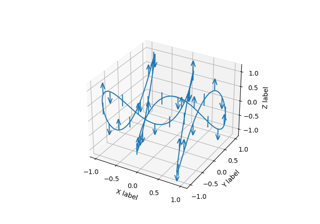3D errorbars

3D errorbars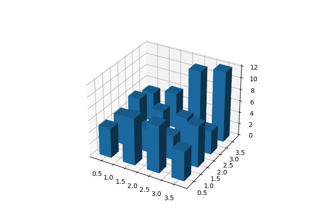Create 3D histogram of 2D data

Create 3D histogram of 2D data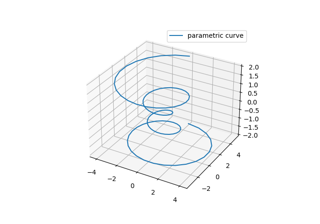Parametric Curve

Parametric Curve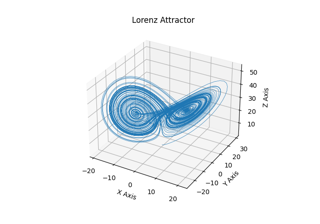Lorenz Attractor

Lorenz Attractor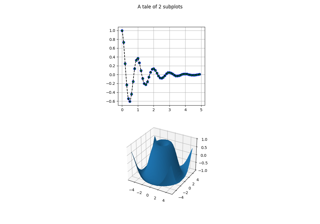2D and 3D Axes in same Figure

2D and 3D *Axes* in same *Figure*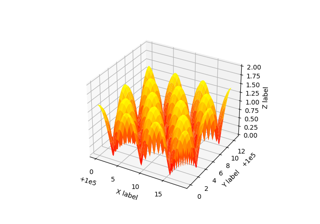Automatic Text Offsetting

Automatic Text Offsetting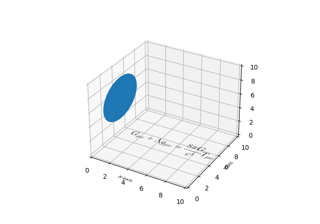Draw flat objects in 3D plot

Draw flat objects in 3D plot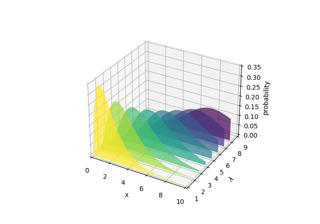Generate polygons to fill under 3D line graph

Generate polygons to fill under 3D line graph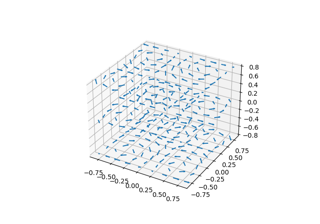3D quiver plot

3D quiver plot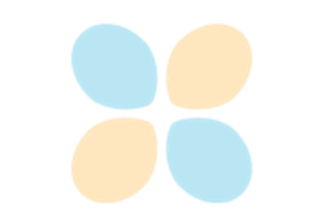Rotating a 3D plot

Rotating a 3D plot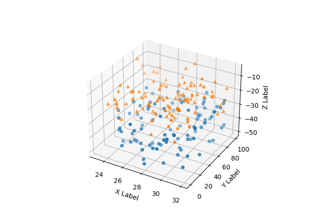3D scatterplot

3D scatterplot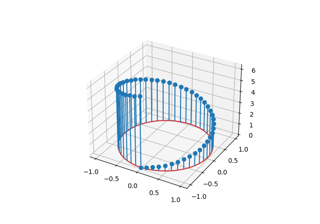3D stem

3D stem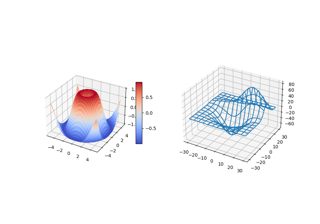3D plots as subplots

3D plots as subplots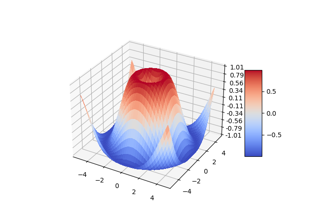3D surface (colormap)

3D surface (colormap)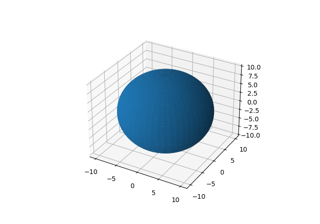3D surface (solid color)

3D surface (solid color)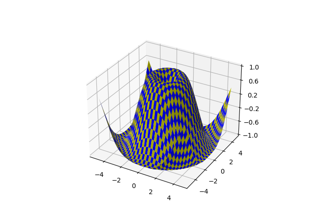3D surface (checkerboard)

3D surface (checkerboard)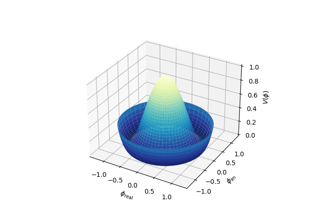3D surface with polar coordinates

3D surface with polar coordinates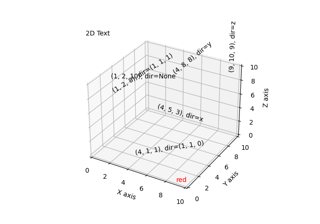Text annotations in 3D

Text annotations in 3D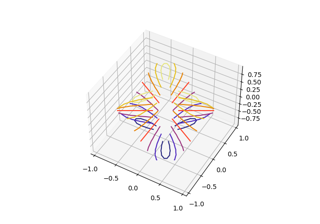Triangular 3D contour plot

Triangular 3D contour plot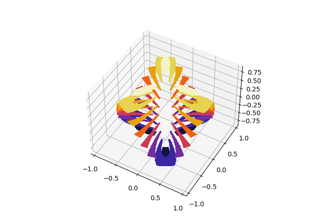Triangular 3D filled contour plot

Triangular 3D filled contour plot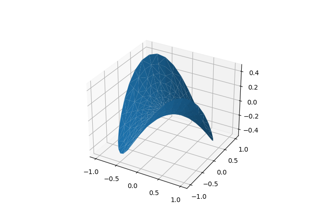Triangular 3D surfaces

Triangular 3D surfaces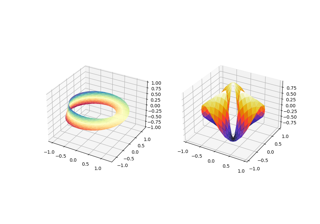More triangular 3D surfaces

More triangular 3D surfaces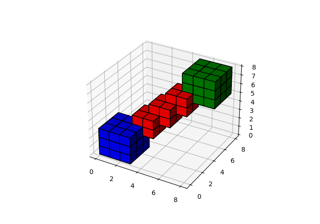3D voxel / volumetric plot

3D voxel / volumetric plot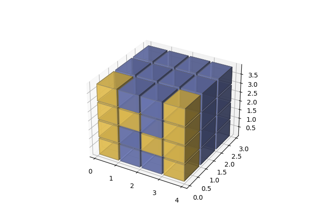3D voxel plot of the numpy logo

3D voxel plot of the numpy logo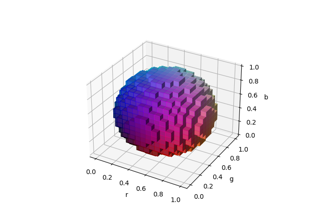3D voxel / volumetric plot with rgb colors

3D voxel / volumetric plot with rgb colors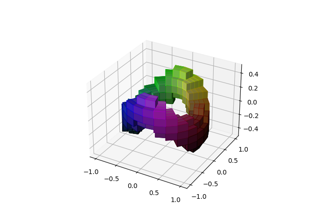3D voxel / volumetric plot with cylindrical coordinates

3D voxel / volumetric plot with cylindrical coordinates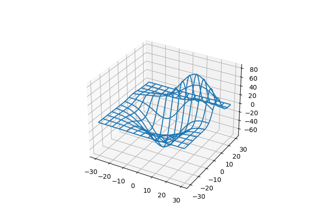3D wireframe plot

3D wireframe plotRotating 3D wireframe plot

Rotating 3D wireframe plot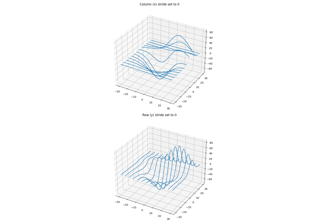3D wireframe plots in one direction

3D wireframe plots in one direction